#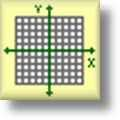Graph Paper

## Printable Math Graph Paper

Here is a graphic preview for all of the graph paper available on the site. You can select different variables to customize the type of graph paper that will be produced. We have Standard Graph Paper that can be selected for either 1/10 inch, 1/4 inch, 3/8 inch, 1/2 inch or 1 centimeter scales. The Coordinate Plane Graph Paper may be selected for either single or four quadrants paper. The Single Quadrant graph paper has options for one grid per page, two per page, or four per page. The Four Quadrant graph paper can produce either one grid per page or four grids per page. The Polar Coordinate Graph Paper may be produced with different angular coordinate increments. You may choose between 2 degrees, 5 degrees, or 10 degrees. We have horizontal and vertical number line graph paper, as well as writing paper, notebook paper, dot graph paper, and trigonometric graph paper.

## Quick Link for All Graph Paper

Click the image to be taken to that Graph Paper.

##### StandardGraph Paper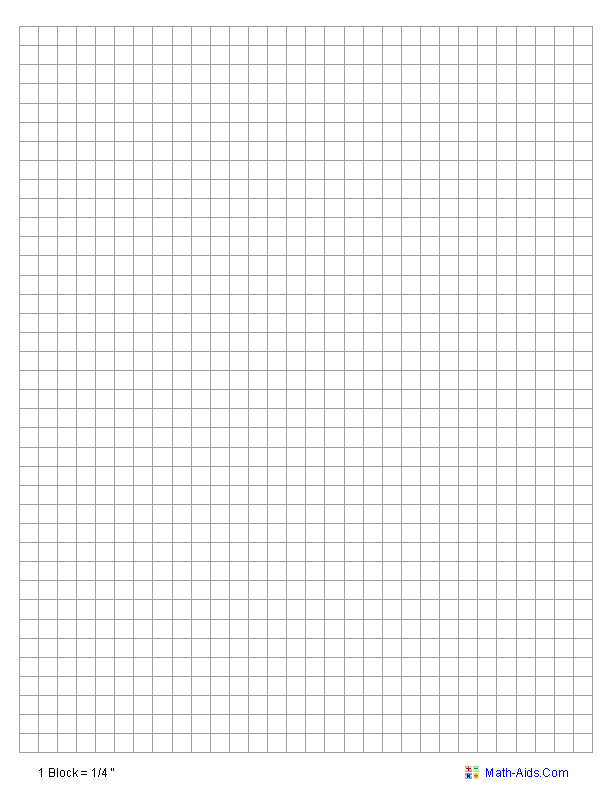##### Single Quadrant 1 Per PageGraph Paper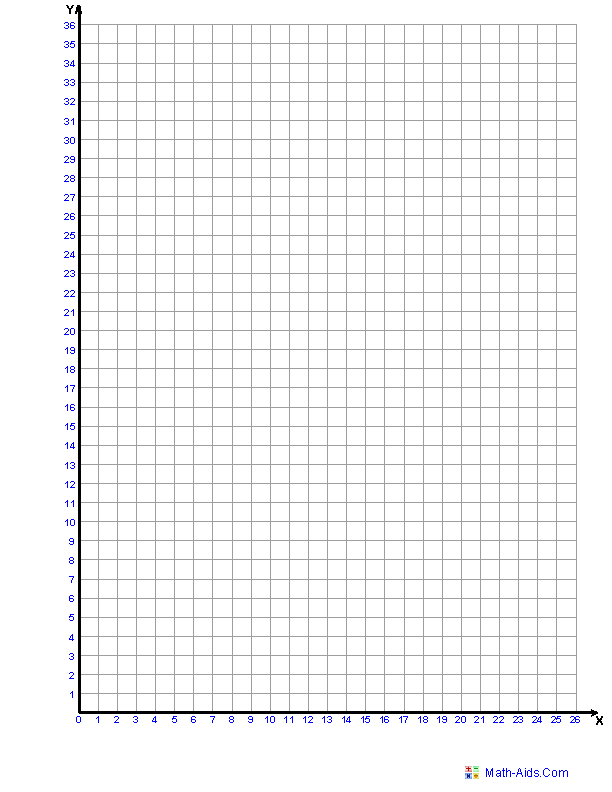##### Single Quadrant 4 Per PageGraph Paper##### Four Quadrant 1 Per PageGraph Paper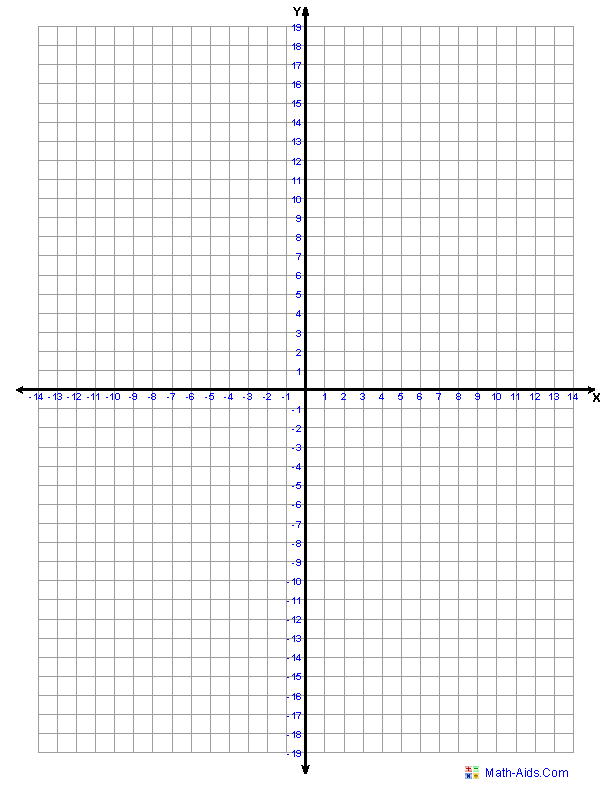##### Four Quadrant 4 Per PageGraph Paper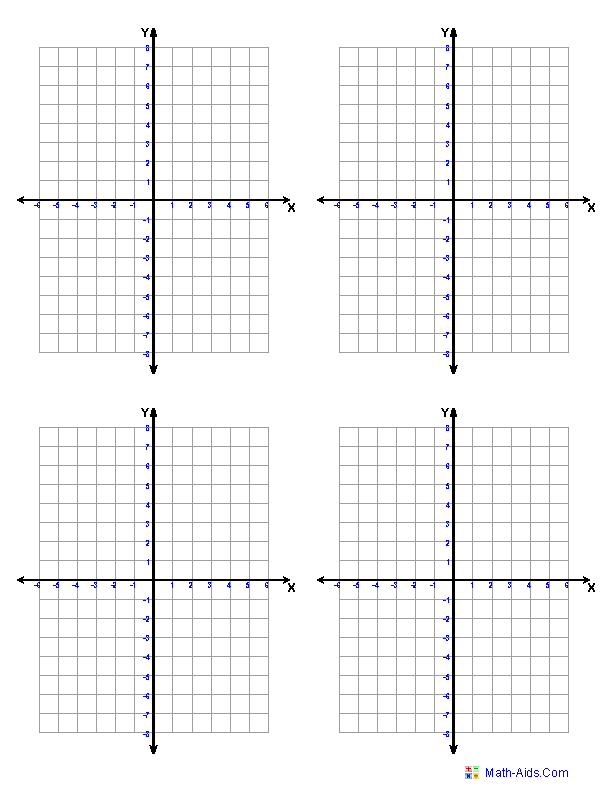##### Four Quadrant 5x5 GridGraph Paper##### Four Quadrant 12 Per PageGraph Paper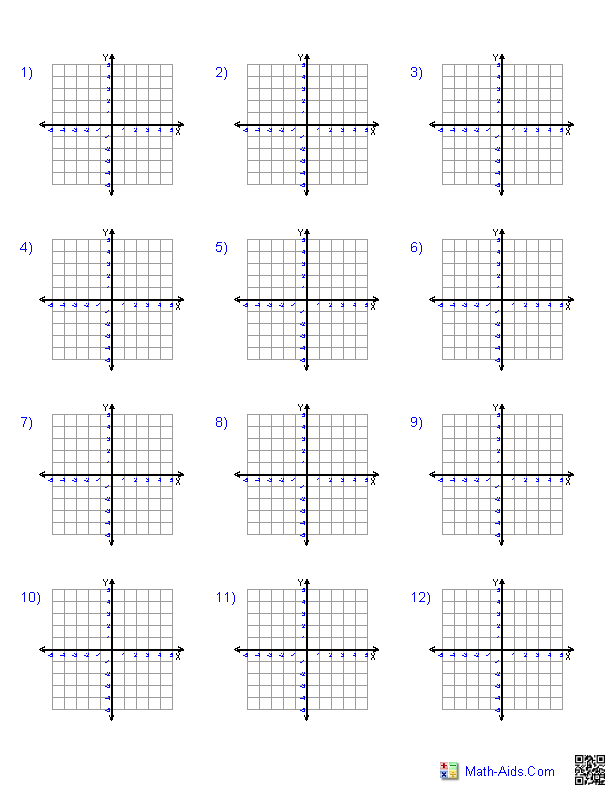##### Polar CoordinateGraph Paper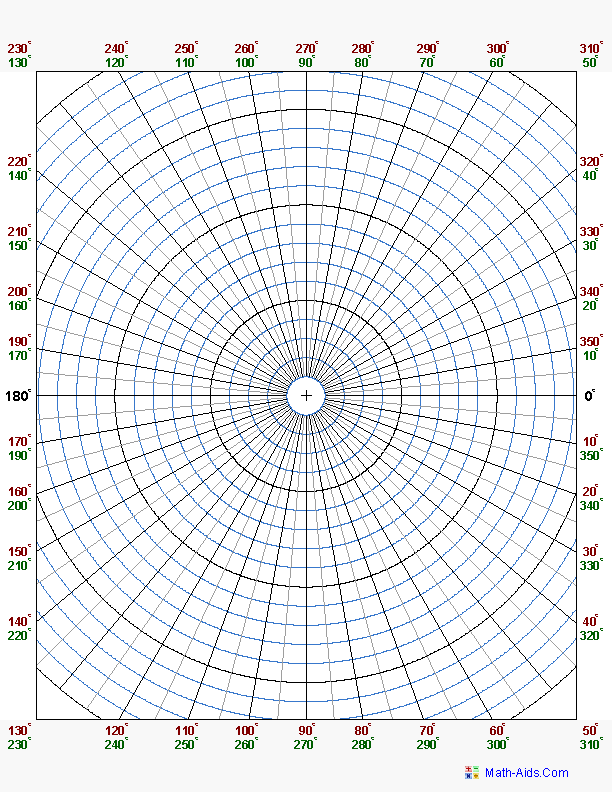##### Logarithmic Graph Paper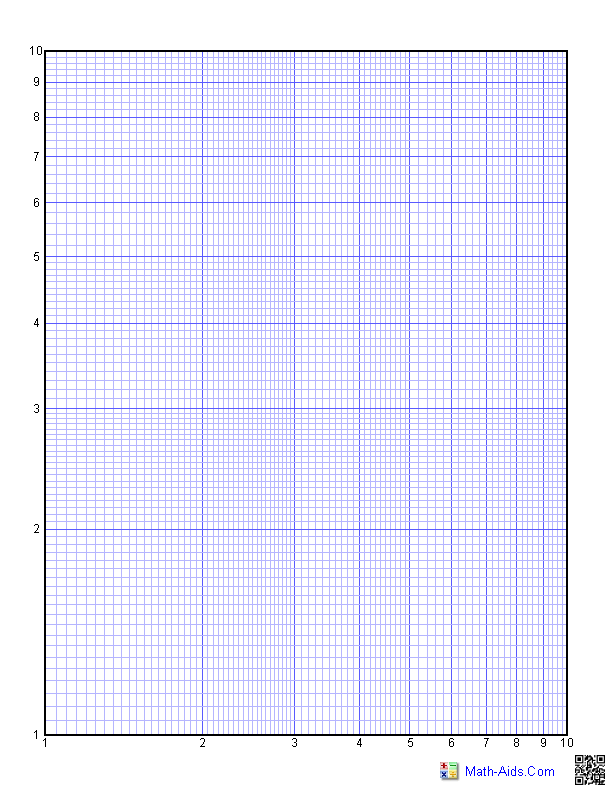##### Horizontal Number LinesGraph Paper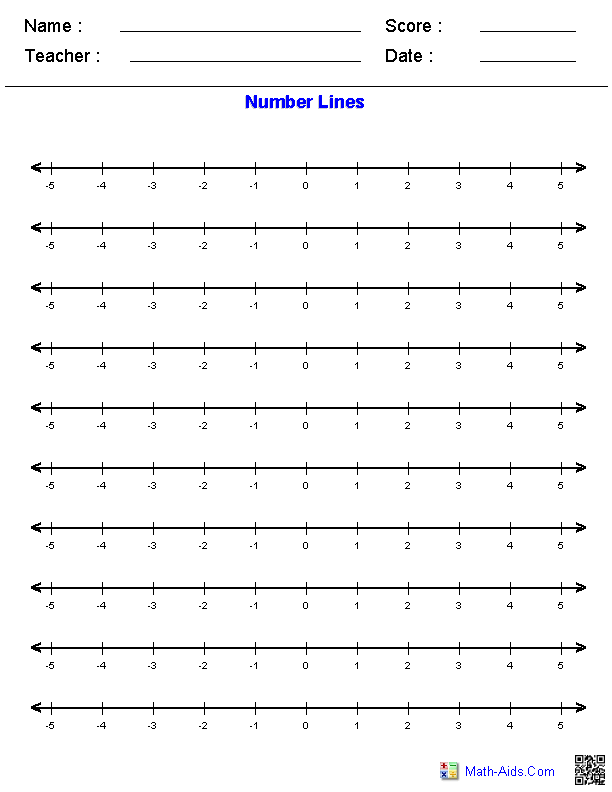##### Vertical Number LinesGraph Paper##### Cornell Notes Template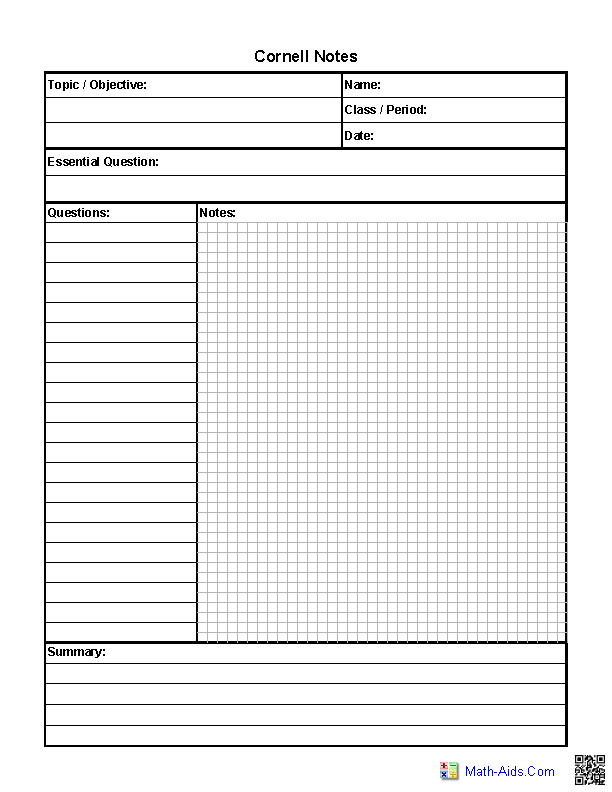##### Writing Paper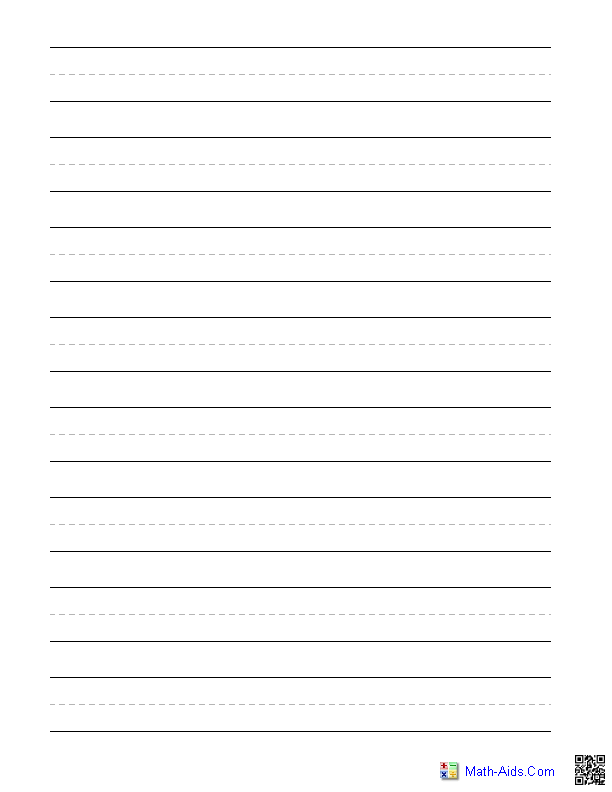##### Notebook Paper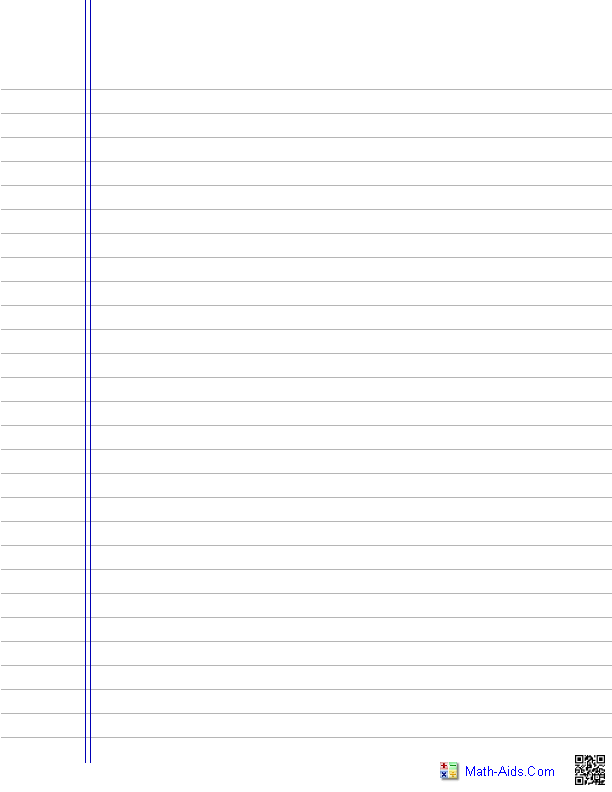##### Dot Graph Paper##### Trigonometric Graph PaperZero to 2 Pi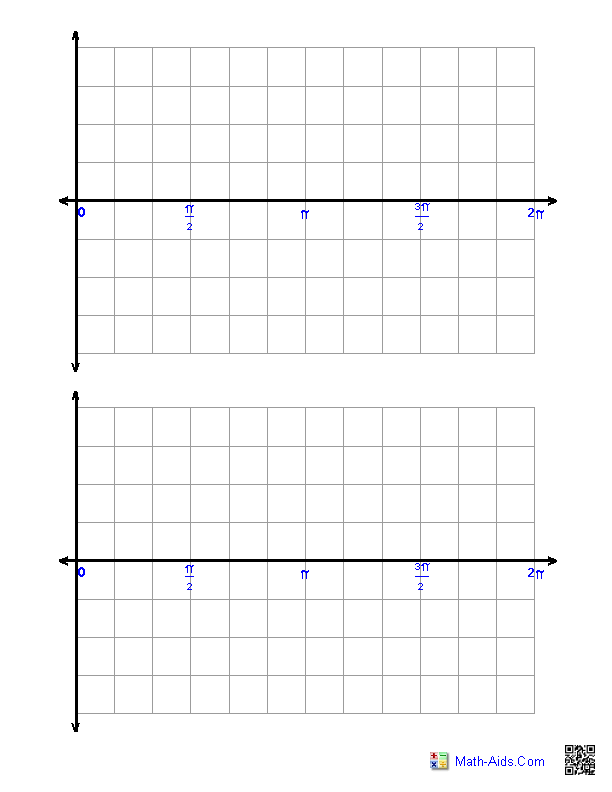##### Trigonometric Graph PaperMinus 2 Pi to Plus 2 Pi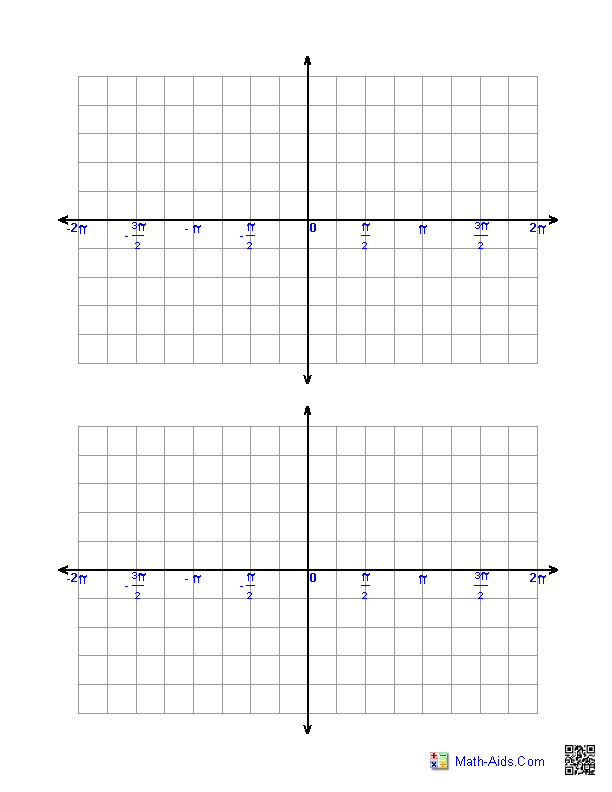Recommended Videos

## Detailed Description for All Graph Paper

Standard Graph Paper
These graph paper generators will produce a blank page of standard graph paper for various types of scales. The available scales are 1/10 inch, 1/4 inch, 3/8 inch, 1/2 inch, and 1 centimeter.

Single Quadrant Coordinate Plane Graph Paper
These graph paper generators will produce a single quadrant coordinate grid for the students to use in coordinate graphing problems. You may select one single quadrant per page, two single quadrants or four single quadrant graphs per page.

Four Quadrant Coordinate Plane Graph Paper
These graph paper generators will produce four quadrant coordinate grids for the students to use in coordinate graphing problems. You may select one full size four quadrant grids per page, or four smaller four quadrant grids per page.

Four Quadrant 5x5 Grid Size Graph Paper
These graph paper generators will produce four quadrant coordinate 5x5 grid size with number scales on the axes on a single page. You may select to the number of graphs per page from 1, 4, 8 or 12.

Four Quadrant Graph Paper (Eight or Twelve per Page)
These graph paper generators will produce four quadrant coordinate grids for the students to use in coordinate graphing problems. You may select eight or twelve four quadrant grids per page.

Polar Coordinate Graph Paper
These graph paper generators will produce a polar coordinate grid for the students to use in polar coordinate graphing problems. You may select different angular coordinate increments to be produced. The different angular increments are 2, 5 and 10 degrees.

Logarithmic Graph Paper
These graph paper generators will produce logarithmic graph paper. You can select different scales for either axis.

Horizontal Number Lines Graph Paper
These graph paper generators will produce a blank page of Horizontal Number Lines for various types of scales. You may enter whole numbers, negative numbers or decimals numbers for the starting and ending numbers. You may select increments that are whole integers or fractions.

Vertical Number Lines Graph Paper
These graph paper generators will produce a blank page of Vertical Number Lines for various types of scales. You may enter whole numbers, negative numbers or decimals numbers for the starting and ending numbers. You may select increments that are whole integers or fractions.

Cornell Notes Template
These Graph Paper generators will produce a Cornell Notes Template. You may select the format of the notes area.

Writing Paper
These graph paper generators will produce a blank page of writing paper for practicing writing letters and numbers.

Notebook Paper
These graph paper generators will produce a blank page of notebook paper.

Dot Graph Paper
These graph paper generators will produce a blank page of dot graph paper.

Trigonometric Graph Paper - Zero to 2 Pi
This Graph Paper generator will produce a blank page of trigonometric graph paper with the x-axis from zero to 2 Pi and two grids per page. You may select the type of label you wish to use for the X-Axis.

Trigonometric Graph Paper - Minus 2 Pi to Plus 2 Pi
This Graph Paper generator will produce a blank page of trigonometric graph paper with the x-axis from Minus 2 Pi to Plus 2 Pi and two grids per page. You may select the type of label you wish to use for the X-Axis.